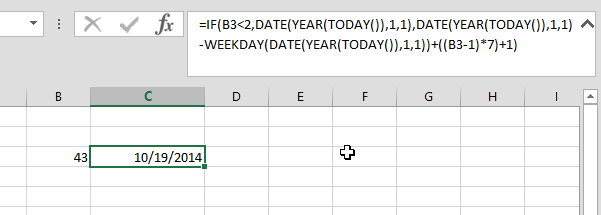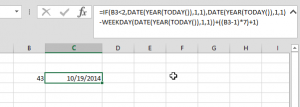This was a request from one of my work managers
She wanted to convert a week number she got in a column into thier dates

Excel already have the WEEKNUM that converts a date into its week number, but the reverse is what needed here

So after my mouth was drooling while she was asking her question, I started a head, and below is the result formula

You just need to place the week number in cell B3, then paste this formula anywhere:

`=IF(B3<2,DATE(YEAR(TODAY()),1,1),DATE(YEAR(TODAY()),1,1)-WEEKDAY(DATE(YEAR(TODAY()),1,1))+((B3-1)*7)+1) `